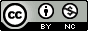## Tuesday, March 8, 2011

### Splitting a Dataset Revisited: Keeping Covariates Balanced Between Splits

In my previous post I showed you how to randomly split up a dataset into training and testing datasets. (Thanks to all those who emailed me or left comments letting me know that this could be done using other means. As things go with R, it's sometimes easier to write a new function yourself than it is to hunt down the function or package that already exists.)

What if you wanted to split a dataset into training/testing sets but ensure that there are no significant differences between a variable of interest across the two splits?

For example, if we use the splitdf() function from last time to split up the iris dataset, setting the random seed to 44, it turns out the outcome variable of interest, Sepal.Length, differs significantly between the two splits.

```splitdf <- function(dataframe, seed=NULL) {
if (!is.null(seed)) set.seed(seed)
index <- 1:nrow(dataframe)
trainindex <- sample(index, trunc(length(index)/2))
trainset <- dataframe[trainindex, ]
testset <- dataframe[-trainindex, ]
list(trainset=trainset,testset=testset)
}

data(iris)
s44 <- splitdf(iris, seed=44)
train <- s1\$trainset
test <- s1\$testset
t.test(train\$Sepal.Length, test\$Sepal.Length)```

What if we wanted to ensure that the means of Sepal.Length, as well as the other continuous variables in the dataset, do not differ between the two splits?

Again, this is probably something that's already available in an existing package, but I quickly wrote another function to do this. It's called splitdf.randomize(), which depends on splitdf() from before. Here, you give splitdf.randomize() your data frame you want to split, and a character vector containing all the columns you want to keep balanced between the two splits. The function is a wrapper for splitdf(). It randomly makes a split and does a t-test on each column you specify. If the p-value on that t-test is less than 0.5 (yes, 0.5, not 0.05), then the loop will restart and try splitting the dataset again. (Currently this only works with continuous variables, but if you wanted to extend this to categorical variables, it wouldn't be hard to throw in a fisher's exact test in the while loop)

For each iteration, the function prints out the p-value for the t-test on each of the variable names you supply. As you can see in this example, it took four iterations to ensure that all of the continuous variables were evenly distributed among the training and testing sets. Here it is in action:

1.P-values to judge balance? When the null hypothesis does hold (mean of training and testing populations are equal), which does hold because of randomization, then the p-value is uniformly distributed over [0,1]. Thus, for a single covariate, the probability you'll have to re-randomize is 1/2; for k covariates, the probability is 1-(1/2)^k. I'm not surprised it took you four tries to get balance based on your criteria.

I think we should appreciate the randomness that occurs, because that is what is allowing us to estimate the out-of-sample error from the testing set.

2.Stephen, could you elaborate on the rationale for choosing p = 0.5 rather than 0.05?

1.My thinking was to make sure there was no remotely significant difference between the columns to randomize on.